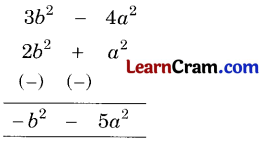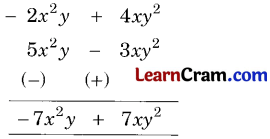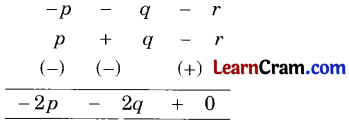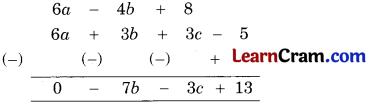# DAV Class 6 Maths Chapter 6 Worksheet 6 Solutions

The DAV Class 6 Maths Solutions and DAV Class 6 Maths Chapter 6 Worksheet 6 Solutions of Introduction to Algebra offer comprehensive answers to textbook questions.

## DAV Class 6 Maths Ch 6 WS 6 Solutions

Question 1.
Subtract:

(a) 3x from 5x
Solution:
3x from 5x = 5x – 3x = 2x

(b) 4a from 3a
Solution:
4a from 3a = 3a – 4a = – a

(c) 13x2 from x2
Solution
13x2 from x2 = x2 – 13x2
= – 12x2

(d) – y from 3y
Solution:
– y from 3y = 3y – (- y)
= 3y + y
= 4y

(e) – 7a from – 3a
Solution:
– 7a from – 3a = – 3a (-7a)
= – 3a + 7a
= 4a

(f) 3abc from – 3 abc
Solution:
3abc from – 3abc = (- 3 abc) – (3abc)
= – 6abcQuestion 2.
Subtract by column method:

(a) 3x + 3y from 2x + 3y
Solution:(b) a2 + 2b2 from 3b2 – 4a2
Solution:(c) 5x2y – 3xy2 from – 2x2y + 4y2
Solution:(d) 2a – 3b + 5 from 5a – b – 2
Solution:(e) p + q – r from – p – q – r
Solution:= – 2p – 2q

(f) a3 + b3 – 3abc from – a3 + b3 + 3abc
Solution:= – 2a3Question 3.
Subtract by horizontal method:

(a) 2x – 3y + 4z from 4x – 6y – z
Solution:
2x – 3y + 4z from 4x – 6y – z
= (4x – 6y – z) – (2x – 3y + 4z)
= 4x – 6y – z – 2x + 3y – 4z
= 2x – 3y – 5z

(b) 2x – 4y from – 4x + 3y
Solution:
2x – 4y from – 4x + 3y
= (- 4x + 3y) – (2x – 4y)
= – 4x + 3y – 2x + 4y
= – 6x + 7y

(c) 5q2 – p2 – 7r from 6p2 – q2 + 2r – 9
Solution:
5q2 – p2 – 7r from 6p2 – q2 + 2r – 9
(6p2 – q2 + 2r – 9) – (5q2 – p2 – 7r)
= 6p2 – q2 + 2r – 9 – 5q2 + p2 + 7r
= (6p2 + p2) + (- q2 – 5q2) + (2r + 7r) – 9
= 7p2 – 6q2 + 9r – 9

(d) – x – y from x + y
Solution:
– x – y from x + y
= (x + y) – (-x – y)
= – x + y + x + y
=> (x + x) + (y + y) = 2x + 2y

(e) 7pqr – 8p + 3 from 10 – 4pqr + 3p
Solution:
7pqr – 8p + 3 from 10 – 4pqr + 3p
= (10 – 4pqr + 3p) – (7pqr – 8p + 3)
= 10 – 4pqr + 3p – 7pqr + 8p – 3
= (10 – 3) + (- 4pqr – 7pqr) + (3p + 8p)
= 7 – 11pqr + 11p

(f) 5x3 + 3x2 – x – 3 from 5x3 – 3x2 + 2x – 3
Solution:
5x3 + 3x2 – x – 3 from 5x3 – 3x2 + 2x – 3
(5x2 – 3x2 + 2x – 3) – (5x3 + 3x22 – x – 3)
= 5x2 – 3x2 + 2x – 3 – 5x3 – 3x2 + x + 3
= – 5x3 + (5x2 – 3x2 – 3x2) + (2x + x) + (- 3 + 3)
= – 5x3 – x2 + 3x + 0
= – 5x3 – x2 + 3xQuestion 4.
Subtract 3x + 2y – 5xy from 0.
Solution:
0 – (3x + 2y – 5xy)
= 0 – 3x – 2y + 5x.y
= – 3x – 2y + 5 xy

Question 5.
From the sum of x2 + x + 1 and x2 – x + 1, subtract – x2 – x + 1.
Solution:
[(x2 + x + 1) + (x2 – x + 1)] – (- x2 – x + 1)
= (x2 + x + 1 + x2 – x + 1) – (- x2 – x + 1)
= x2 + x + 1 + x2 – x + 1 + x2 + x – 1
= (x2 + x2 + x2) + (x – x + x) + (1 + 1 – 1)
= 3x2 + x + 1Question 6.
Subtract the sum of 2a + 3b, a – 2b + c, – a + 2c and 4a + 2b – 5 from 6a – 4b + 8.
Solution:
First we find the sum of 2a + 3b, a – 2b + c, – a + 2c and 4a + 2b – 5.
= (2a + 3b) + (a – 2b + c) + (- a + 2c) + (4a + 26-5)
= 2a + 3b + a – 2b + c – a + 2c + 4a + 26-5
= (2a + a – a + 4a) + (3b – 2b + 2b) + (c + 2c) – 5
= 6a + 3b + 3c – 5
Now subtract 6a + 3b + 3c – 5 from 6a – 4b + 8
= 6a – 4b + 8= – 7b – 3c + 13